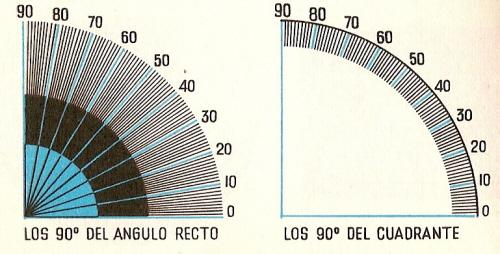Measurement of angles to printName: ______________________________________  Subject: _______________________ Date: _______

Write the missing word

1. The right angle It measures 90░ (degrees), the straight angle measures 180░ and the full angle measures 360░. Thus, the degree is 1/90 of the right angle, 1/180 of the straight angle or 1/360 of the full angle.

 The straight angle has... The right angle has... The full angle has......2. The protractor

It is an instrument used to measure angles. It is typically made of transparent plastic or glass with a fixed point (0) in the center of the base and an arc of 180║. It is used to measure a given angle and draw an angle of a specified number of degrees. The vertex of the angle should coincide with the point 0 of the protractor.

Example: the angle XOY measures 42║.

 The angle of 42░ is... The angle of 140║ is... The angle of 90░ is... The angle of 89░ is... The angle of 91║ is...3. Complementary Angles
Two Angles are Complementary if they add up to 90 degrees (right angle). The angle 1 and 2 are complementary because they add up to 90░.

 The angle 1 is... The angle 2 is... The sum 1 + 2 is...4. Supplementary Angles
Two Angles are supplementary if they add up to 180 degrees░ (straight angle). In the picture, we see that the sum of the angles 3 and 4 make a straight angle of 180 degrees.

 The angle 3 is... ... The angle 4 is... The sum 3 + 4 is...5. Vertically opposite angles
Those that have the same vertex and their sides are opposite rays. In the picture, the angles a and c, and b and d are opposite.
These angles are equal, that means, a = c and b = d. We also note that a and b are supplementary and measure 180 ║. c and b are also supplementary. As the common sum is b, we have a = c.

Look the above picture and answer:

 The angles a and b are... ... The angles a and c are...... The angles a and d are... The angles b and c are... The corners b and d are... The angles b and a are...

| Educational applications | Mathematics |
In Spanish  | Interactive

«Arturo Ramo GarcĒa.-Record of intellectual property of Teruel (Spain) No 141, of 29-IX-1999
Plaza Playa de Aro, 3, 1║ DO 44002-TERUEL